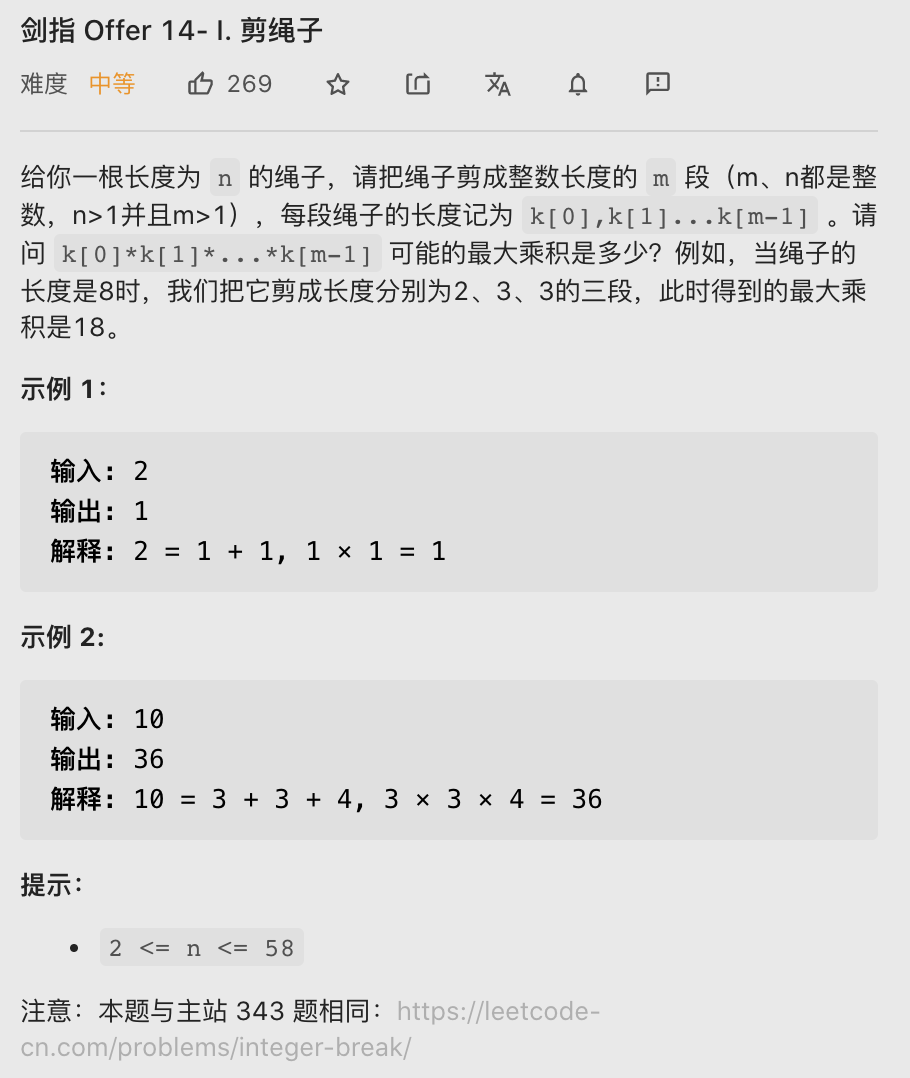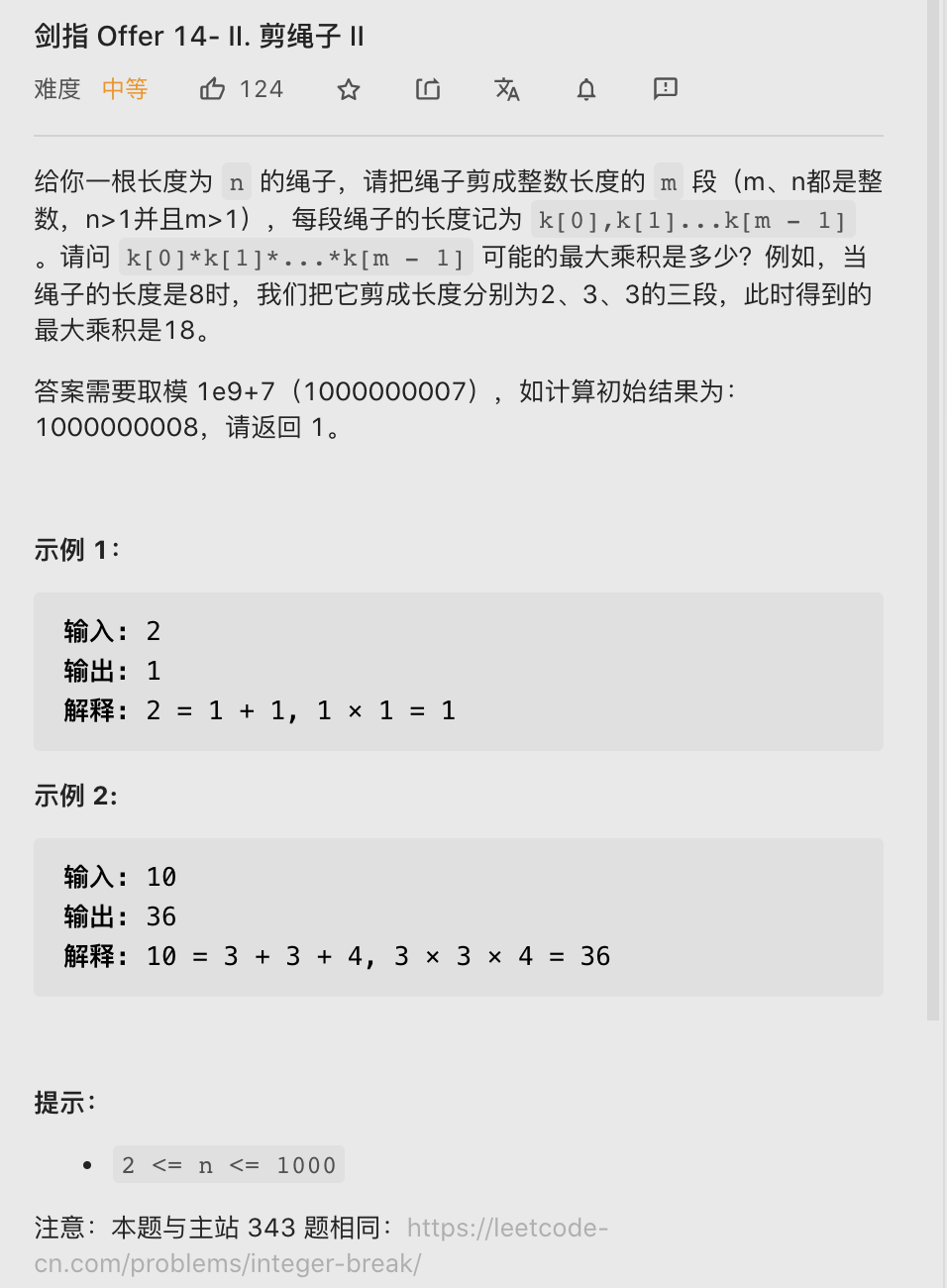# 【LeetCode笔记】剑指 Offer 14. 剪绳子 I II（Java、动态规划、偏数学）

2021/7/20 18:24:03 浏览：

# 剪绳子 I

### 题目描述

• 还是比较偏数学的一道题，通过获取结论来获得最优解### 思路 && 代码

##### 1. 动态规划 O( n 2 n^2 )、O(n)
• dp[i]：长度为 i 的绳子，可达到的最大值
• 状态转移方程：以之前的值，循环组合，找出可取的最大值
class Solution {
public int cuttingRope(int n) {
if(n <= 3) {
return n - 1;
}
int[] dp = new int[n + 1];
// 1. 初始化
dp = 1;
dp = 2;
dp = 3;

for(int i = 4; i <= n; i++) {
int max = 0;
for (int j = 1; j <= i / 2; j++) {
// 2. 状态转移方程
max = Math.max(max, dp[j] * dp[i - j]);
}
dp[i] = max;
}
return dp[n];
}
}

##### 2. 最优解：数学方法 O(n)、O(1)
• 见注释的正确性证明
• 主要做法就是循环地切出3，直到所剩值不大于4为止（ n == 4 时候，直接用是最好的，因为 x * 4 == x* 2 * 2 > x * 3 * 1）
class Solution {
public int cuttingRope(int n) {
// 数学方法：O(n) & O(1)
if(n < 4) {
return n - 1;
}
int res = 1;
// 循环切除3
while(n > 4) {
res *= 3;
n -= 3;
}
// 最后乘一次 1 or 2 or 3 or 4
return res * n;
}
}
/*
* 正确性证明（参考评论区大佬）
* 1. 任何大于1的数都可由 2 & 3 组成（偶数可2累加，奇数就是前一个偶数加一次3）
* 2. 2 * 2 == 1 * 4 ，且 2 * 3 > 1 * 5，因此拆成2 & 3得到积最大
* 3. 因为 2 * 2 * 2 < 3 * 3，因此拆成 3 性价比最高
*/


# 剪绳子 II

### 题目描述

• 相较于1，多了一个取模操作### 思路 && 代码

class Solution {
public int cuttingRope(int n) {
if(n < 4) {
return n - 1;
}
long res = 1L;
while(n > 4) {
res *= 3;
res %= 1000000007;
n -= 3;
}
return (int)(res * n % 1000000007);
}
}